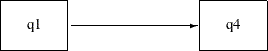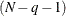### Example 29.6 Linear Regression Model

This example shows how you can use PROC CALIS to fit the basic regression models. Unlike the preceding examples ( Example 29.1, Example 29.2, Example 29.3, and Example 29.4) where you specify the covariance structures directly, in this example the covariance structures being analyzed are implied by the functional relationships specified in the model. The PATH modeling language introduced in the current example requires you to specify only the functional or path relationships among variables. PROC CALIS analyzes the implied covariance structures that are derived from the specified functional or path relationships.

Consider the same `sales` data as in Example 29.1. This example demonstrates a simple linear regression that uses `q1` (the sales in the first quarter) to predict `q4` (the sales in the fourth quarter).

In covariance structural analysis, or in general structural equation modeling, relationships among variables are usually represented by the so-called path diagram. For example, you can represent the linear regression of `q4` on `q1` by the following simple path diagram:

Output 29.6.1:In the path diagram, `q1` is an exogenous (or independent) variable and `q4` is an endogenous (or dependent) variable. Formally, a variable in a path diagram is endogenous if there is at least one single-headed arrow pointing to it. Otherwise, the variable is exogenous. In some situations, researchers apply causal interpretations among variables in the path diagram, with the single-headed arrows indicating the causal directions. However, causal interpretations are not a requirement for using covariance structure analysis or structural equation modeling.

It is easy to transcribe the preceding path diagram into the PATH model specification in PROC CALIS, as shown in the following statements:

```proc calis data=sales;
path   q1  ===>  q4;
run;
```

Output 29.6.2 shows the modeling information of the linear regression model. It shows that all 14 observations are used and the model type is PATH. PROC CALIS analyzes the (implied) covariance structure model for the data. In the next table of Output 29.6.2, PROC CALIS shows the nature of the variables in the model: `q4` is an endogenous manifest variable and `q1` is an exogenous manifest variable. There is no latent variable in this simple path model.

Output 29.6.2: Modeling Information of the Linear Regression Model for the `Sales` Data

 Simple Linear Regression Model by the PATH Modeling Language

The CALIS Procedure
Covariance Structure Analysis: Model and Initial Values

Modeling Information
Maximum Likelihood Estimation
Data Set WORK.SALES
N Records Read 14
N Records Used 14
N Obs 14
Model Type PATH
Analysis Covariances

Variables in the Model
Endogenous Manifest q4
Latent
Exogenous Manifest q1
Latent

Output 29.6.3 shows the initial model specification. The path is in the first table. A parameter name is attached to the path. The name `_Parm1`, which is generated automatically by PROC CALIS, denotes the effect parameter of `q1` on `q4`. In the context of linear regression, `_Parm1` also denotes the regression coefficient.

Output 29.6.3: Initial Specification of the Linear Regression Model for the `Sales` Data

Initial Estimates for PATH List
Path Parameter Estimate
q1 ===> q4 _Parm1 .

Initial Estimates for Variance Parameters
Variance
Type
Variable Parameter Estimate
Exogenous q1 _Add1 .
Error q4 _Add2 .

Next, Output 29.6.3 shows the variance parameters in the model. You do not need to specify any of these parameters in the preceding PATH model specification—because PROC CALIS adds these parameters by default. `_Add1` denotes the variance parameter for the exogenous variable `q1`. `_Add2` denotes the error variance parameter for the endogenous variable `q4`.

In the PATH model of PROC CALIS, all variances of exogenous variables and all error variances of endogenous variables are free parameters by default. In most practical applications, these parameters are usually free parameters in models and it would be laborious to specify them each time when you fit a covariance structure model. Therefore, to make the PATH model specification more efficient and easier, PROC CALIS sets these free parameters by default. In fact, with these default parameters in the PATH model, PROC CALIS produces essentially the same regression analysis results as those produced by common linear regression procedures such as PROC REG. This consistency is shown in the subsequent estimation results for the current example.

You can also explicitly specify those otherwise default parameters of the PATH model in PROC CALIS. Depending on the modeling situation, you can set any parameter in the PATH model as a free, fixed, or constrained parameter. You can also provide names for the parameters. Naming parameters is very useful for parameter referencing and for setting up parameter constraints. See Example 29.4. For details, see the PATH statement and the section The PATH Model.

Output 29.6.4 shows some fit statistics from the linear regression model. The model fit chi-square is 0 with 0 degrees of freedom. This is a perfect model fit. The fit is perfect because the covariance model contains three distinct elements (variance of `q1`, variance of `q4`, and covariance between `q1` and `q4`) that are fitted perfectly by three parameters: `_Parm1` for the effect of `q1` on `q4`, `_Add1` for the variance of variable `q1`, and `_Add2` for the error variance of variable `q4`. Thus, the unconstrained linear regression model estimates are simply a transformation of the covariance elements. Hence, the model is saturated with a perfect fit and zero degrees of freedom.

Output 29.6.4: Model Fit of the Linear Regression Model for the `Sales` Data

Fit Summary
Chi-Square 0.0000
Chi-Square DF 0
Pr > Chi-Square .
Standardized RMR (SRMR) 0.0000
RMSEA Estimate .

Output 29.6.5 shows the estimates of the model. The effect of `q1` on `q4` is 0.6544 (standard error=0.7571). The associated t value is 0.86433, which is not significantly different from zero. The estimated variance of `q1` is 0.3383 and the estimated error variance for `q4` is 2.5207. Both estimates are significant.

Output 29.6.5: Parameter Estimates of the Linear Regression Model for the `Sales` Data

PATH List
Path Parameter Estimate Standard
Error
t Value
q1 ===> q4 _Parm1 0.65436 0.75707 0.86433

Variance Parameters
Variance
Type
Variable Parameter Estimate Standard
Error
t Value
Exogenous q1 _Add1 0.33830 0.13269 2.54951
Error q4 _Add2 2.52066 0.98869 2.54951

For a simple linear regression such as this one, you could have used PROC REG. You get essentially the same estimates by specifying the following statements:

```proc reg data=sales;
model q4 = q1;
run;
```

Output 29.6.6 shows the parameter estimates from PROC REG. The intercept estimate is 2.7604 (standard error=1.1643) and the regression coefficient is 0.6544 (standard error=0.7880). The regression coefficient estimate matches PROC CALIS. However, the corresponding standard error estimate in PROC CALIS is 0.7571, which is slightly different from PROC REG. This difference is due to the different variance divisors that are used in calculating the standard error estimates. PROC CALIS uses (N – 1) as the divisor (by default) while PROC REG uses, where N is the number of observations and q is the number of regression coefficients. In the current example, q is 1 so that the variance divisor in PROC REG is 1 less than the divisor in PROC CALIS. If you have at least a moderate sample size and the number of regression parameters is relatively small compared to the sample size, the discrepancy due to using different variance divisors is of little consequence.

Output 29.6.6: Parameter Estimates from PROC REG for the `Sales` Data

Parameter Estimates
Variable DF Parameter
Estimate
Standard
Error
t Value Pr > |t|
Intercept 1 2.76040 1.16430 2.37 0.0353
q1 1 0.65436 0.78798 0.83 0.4225

By default, PROC CALIS analyzes only the covariance structures, which are properties of the second-order moments of the data. PROC CALIS does not automatically produce intercept estimates, which are properties of the first-order moments of the data.

In order to produce the intercept estimate in the linear regression context, you can add the MEANSTR (mean structures) option in the PROC CALIS statement, as shown in the following statements:

```proc calis data=sales meanstr;
path   q1  ===>  q4;
run;
```

Output 29.6.7 shows the parameter estimates of the model with the MEANSTR option added. Compared with Output 29.6.5, Output 29.6.7 produces one more table: estimates of the mean and intercept. The intercept estimate for `q4` is 2.7604, which matches the intercept estimate from PROC REG. The estimated mean of `q1` is 1.3671. All other estimates are the same for the analyses with and without the MEANSTR option.

Output 29.6.7: Parameter Estimates of the Linear Regression Model with the MEANSTR option for the `Sales` Data

PATH List
Path Parameter Estimate Standard
Error
t Value
q1 ===> q4 _Parm1 0.65436 0.75707 0.86433

Variance Parameters
Variance
Type
Variable Parameter Estimate Standard
Error
t Value
Exogenous q1 _Add1 0.33830 0.13269 2.54951
Error q4 _Add2 2.52066 0.98869 2.54951

Means and Intercepts
Type Variable Parameter Estimate Standard
Error
t Value
Intercept q4 _Add3 2.76040 1.12480 2.45413
Mean q1 _Add4 1.36714 0.16132 8.47491

Linear regression estimates from PROC CALIS are comparable to those obtained from PROC REG, although the two procedures have different default treatments of the variance divisor in calculating the standard error estimates. With the MEANSTR option in the PROC CALIS statement, you can analyze the mean and covariance structures simultaneously. PROC CALIS prints the estimates of the intercepts and means when you model the mean structures.

This example shows how you can fit the linear regression model as a PATH model in PROC CALIS. You need to specify only path relationships among the variables in the PATH statement, because the implied covariance structures are generated and analyzed by PROC CALIS. To make model specification more efficient, PROC CALIS sets default variance parameters for exogenous variables and default error variance parameters for endogenous variables. You can also overwrite these default parameters by explicit specifications. See Example 29.7 for some sophisticated regression models that you can specify with PROC CALIS. See Example 29.17 for a more elaborate path model specification.